Browse by Topic
Related Topics

# Balancing Rotating Machinery with Wolfram SystemModeler

Explore the contents of this article with a free Wolfram SystemModeler trial. One of the most common causes for vibrations in mechanical systems is imbalance in the rotating parts of a machine. Much effort has therefore gone into developing methods and devices for balancing rotating machines.

Balance is a requirement for many types of rotating machinery, such as electric motors, pumps, fans, turbines, generators, centrifugal compressors, and propellers. Many people know about the balance of their car wheels. If these systems are not properly balanced, the vibration will cause not only reduced efficiency and component fatigue but also disturbances for the environment, such as vibration and noise. The most common methods for balancing rotating machinery are the influence coefficient method and the modal balancing method. The car wheel balancing is, for instance, a subpart of the influence coefficient method.

Wolfram SystemModeler is used for modeling the rotor, and the Wolfram Language for the evaluation of the results. The workflow shows how powerful it is to combine these two softwares.

A disc with mass m is mounted on a shaft with stiffness k. The rotor rotates with the angular velocity W. The disc has an imbalance u. The unit for the imbalance is kg*m.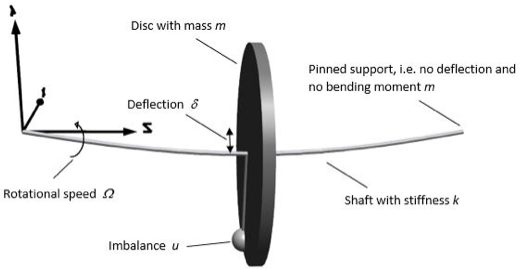The deflection of the shaft from its rest position will be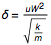.

A resonance occurs at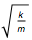. To eliminate the vibration, all you have to do is mount an equal imbalance opposite the existing one. However, in reality it is not that simple. There may be more than one disc. It is often not possible to put the balancing weight on an arbitrary position. You only have certain axial positions, called balancing planes, to work with. In, for instance, a generator or gas turbine, you cannot open up a system and mount weights inside a closed compartment. You most likely need to put the balancing weight close to the bearings. A more realistic example is shown in the first film. It consists of a shaft that carries a flywheel and a gear, along with two smaller discs for balancing. Both the discs and flywheel have an imbalance. Resonance occurs at close to 25 seconds into the film. (Note that the deflections have been scaled 10x).

#### Influence coefficient method, basic equations

In film one, it is not possible to place an equal imbalance opposite the existing one in order to balance the rotor. This is due to two reasons. The first is that the imbalance is not known. The second is that it is not possible to put extra masses on the disc and flywheel. This is a very common situation in real applications. Most parts of a rotor are typically not reachable after mounting. Instead, the balancing engineer needs to work with balancing planes that are normally closed to the bearings.

In the example, both the discs and flywheel have an imbalance. This is illustrated with a mass on the outer diameter and denoted as v1 and v2 in the figure below. Neither the size nor positions of them are known. There are also two balancing planes, u1 and u2. With two balancing planes, it is possible to correct both a static and dynamic imbalance: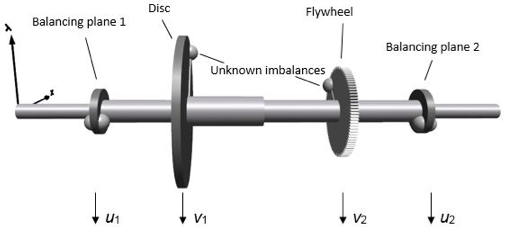Now, the influence coefficient method is rather straightforward. The vibrations are measured in two different locations, v1 and v2. In this example we measure directly on the discs, but it is more realistic to measure on the bearings. The aim here is to show how the deflections of the discs can be reduced. The vibrations can be measured with displacements, velocities, or acceleration. For the basic principle, it doesn’t matter which one of the measuring methods is used, but in reality the accuracy of the results is dependent on the measuring method. For higher frequencies, measuring acceleration is preferred; for lower frequencies, velocities or even displacements may be a better choice.

The imbalances u1 and u2 are known weights mounted on a measured position (radius and angle).

Both u and v are consequently complex variables, which means that they describe both amplitude and phase. Assuming that the system is linear, the vibration of the rotor can then be described as:

v1 = r11 u1+ r12 u2 + v10

v2 = r21 u1+ r22 u2 + v20

where v10 and v20 are the initial vibrations. In matrix form: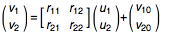Or:

v = R u +v0,

where R is the receptance of the system. The aim of the balancing is, of course, to eliminate or at least minimize the vibrations v1 and v2.

The procedure is as follows.

1) Run the system without the balancing weights, i.e. u1 = u2 = 0. The measurement will give v10 and v20.

2) Apply a test weight at one of the balancing planes—for instance, u1 t. The size and direction don’t matter. The measurement now gives v11 and v21:

v11 = r11 u1 t + v10
v21 = r21 u2 t + v20,

which gives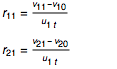3) Remove u1 t and apply a new test weight u2 t at the second balancing plane. In the same way as above: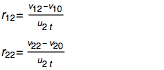4) We now know R and v0. We want the vibration v = 0. This can, at least in theory, easily be fixed by choosing

u = R-1 v0

Apply these balancing weights, and the vibration will be zero.

#### Wolfram SystemModeler model

The SystemModeler model is built up with standard components and an Euler–Bernoulli beam. The Euler–Bernoulli beam theory does not take into account shear deformation and rotational inertia effects, making it suitable for describing the behavior of long beams. This is typical when the length of the shaft is three or more times the size of the diameter. For shorter beams, the Timoshenko beam theory is more accurate. The difference in this case is one or a few percent on eigenfrequencies, and less for deflections. In this application, we use 16 beam elements. We need to have a component for external damping close to the mass, since we have used “pinned” support. In reality, external damping will include the bearings: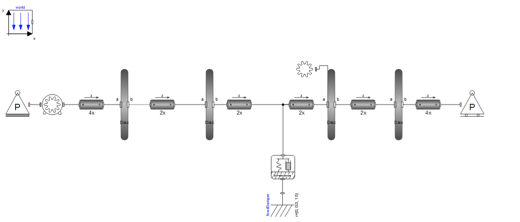The deflection (v1 and v2) of the flywheel (disc 1) and a gear (disc 2) during a startup from 0 to 40 Hz can be seen in the plot below. A resonance close to 25 Hz (= 1500 rpm) is noted. The high vibration could also be seen in film one: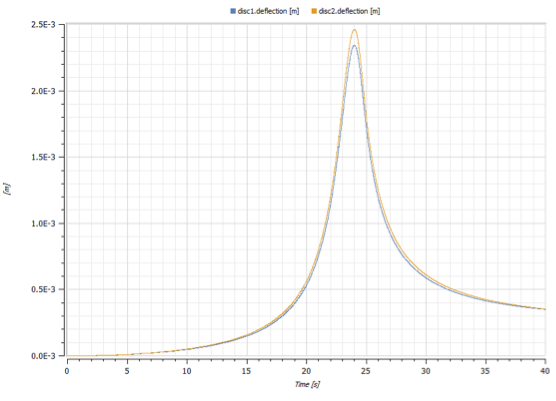The aim now is to reduce this vibration as much as possible with the influence coefficient method, and to do this we combine the Wolfram Language and SystemModeler.

#### Wolfram Language code

Initialize the link between the Wolfram Language and SystemModeler. Set up the working directory and choose the correct model: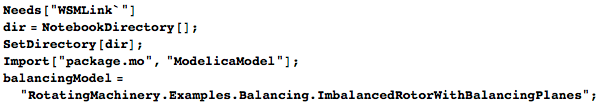Run three different simulations for 40 seconds: the first one without any added test imbalance; the second with 2·0.05 kg m^2 at imbalance plane #1; and the third with 2·0.05 kg m2 at imbalance plane #2. In the last two cases, the imbalance is applied at 0°: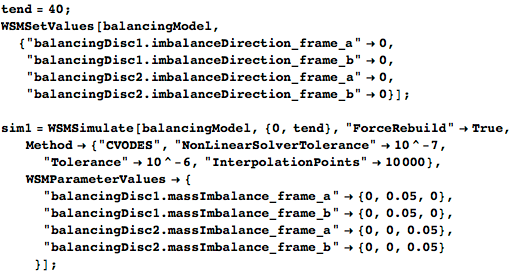Evaluate the phase and deflections for these simulations: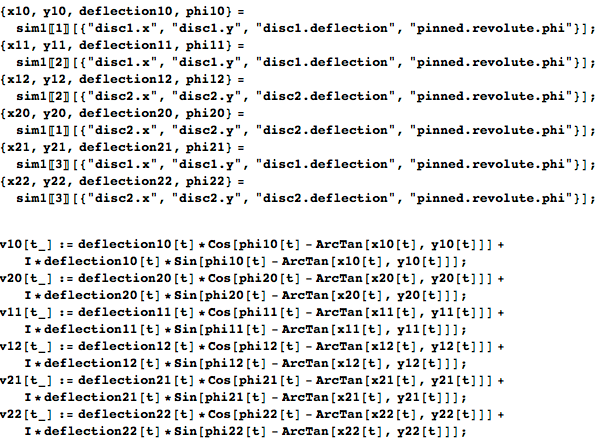The receptance of the system can now be calculated, and after that, the optimum balancing weights and positions can be calculated: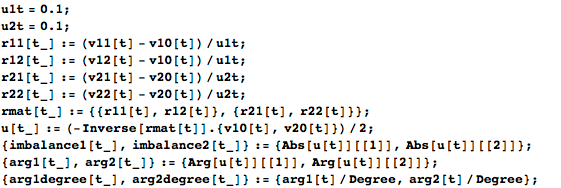Finally, run the model with optimal balancing weights: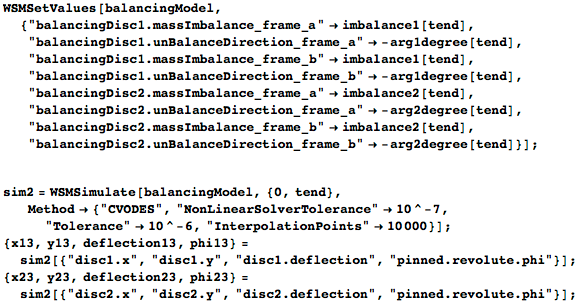#### Results

The rotor used in this example is nonsymmetric in the axial direction, i.e. the masses and the shaft diameters are different. If the system is symmetric, the deflection at 30 Hz after balancing will be less than 0.1%, compared to the deflection before balancing. It is easy to check this by changing the values in the model. Due to the axial asymmetry in this example, does it matter at which speed the balancing is performed? Normally a balancing is preferably performed close to the running speed. With the Wolfram Language, it’s very easy to study this. Simply plot the balancing weights and phases during the run-up: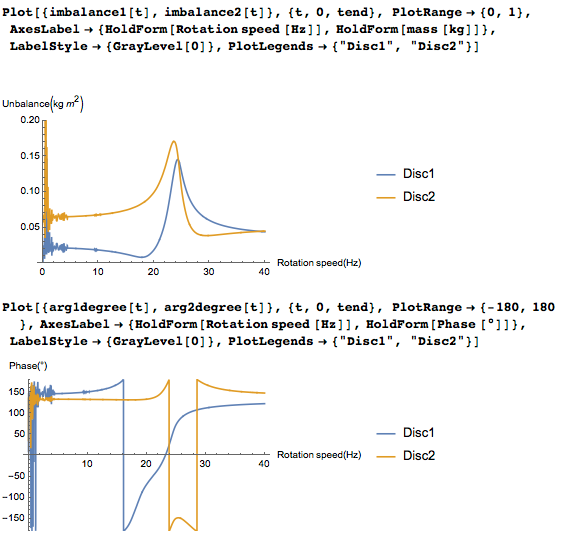As can be seen, it is hard to find balance weights when the rotor rotates close to its resonance speed. It would also be wise to wait a while before measuring when a speed is reached; in reality, you need to wait till the temperature etc. has stabilized. But in this blog, we will skip the waiting time. The optimal balanced rotors’ reduction of the vibration amplitude for disc 1 will be: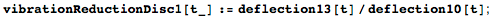And for disc 2 will be: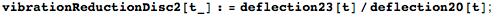Plot the result: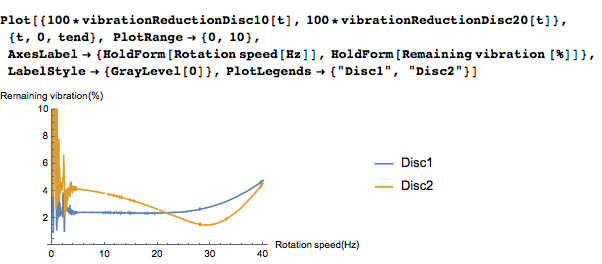From the above figure it can be noted that for this simple case, the vibration will be reduced to around 2%–4% of the original vibration during the run-up. The initial noise before five seconds can be ignored. The system has not yet stabilized, and the total deflection is very low.

Below, the difference can be seen more clearly. The two curves with highest vibration occur before the balancing: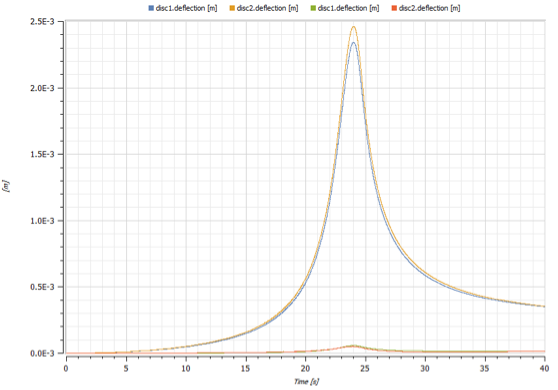The main reason for balancing at the shaft’s operational running speed is that the system normally is more complex than this—for instance, systems with nonlinear supports, more than two bearings, or bent shafts all need to have a stable rotor and (when applicable) oil temperature. Circumstances that give the optimum balancing speed occur at running speed. If the speed varies, a best choice is needed. Exactly how to optimize depends on the application, but with Mathematica a statistical evaluation will be straightforward no matter what approach is chosen.

The behavior after the balancing is shown in the following video. (Note that the deflections have been scaled 10x.)

#### Summary

SystemModeler is a powerful tool for studying advanced problems in rotating machinery. Combined with the Wolfram Language, it gives tremendous opportunities to work with and analyze your models and results. I have shown how rotating machinery can be balanced, and how the balancing speed affects the results. The model can easily be extended to encompass everything from nonlinearities to stochastic sensor noise.

To learn more about what affects the balancing results, I recommend playing around with a model like this one. For instance, will the vibration reduce even further if the “balanced rotor” is balanced once again? If we had used the balancing weights from, say, 5 Hz, what would the vibration at 40 Hz become? How does signal noise affect the results? There are many more or less intelligent balancing methods besides the influence coefficient method and modal balancing method. Try one of those and learn how it works.